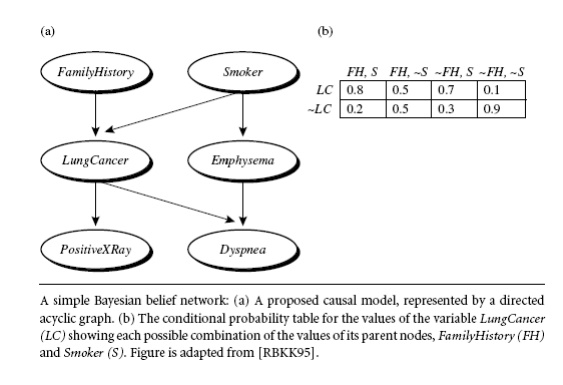Home | | Data Ware Housing and Data Mining | Bayesian Classification

# Bayesian Classification

“What are Bayesian classifiers?” Bayesian classifiers are statistical classifiers. They can predict class membership probabilities, such as the probability that a given tuple belongs to a particular class.

Bayesian Classification

“What are Bayesian classifiers?” Bayesian classifiers are statistical classifiers. They can predict class membership probabilities, such as the probability that a given tuple belongs to a particular class.

Bayesian classification is based on Bayes’ theorem, a simple Bayesian classifier known as the naïve Bayesian classifier Bayesian classifiers have also exhibited high accuracy and speed when applied to large databases.

1. Bayes’ Theorem

. Let X be a data tuple. In Bayesian terms, X is considered ―evidence.‖ As usual, it is described by measurements made on a set of n attributes. Let H be some hypothesis, such as that the data tuple X belongs to a specified class C. For classification problems, we want to determine P(HjX), the probability that the hypothesis H holds given the ―evidence‖ or observed data tuple X. In other words, we are looking for the probability that tuple X belongs to class C, given that we know the attribute description of X.

“How are these probabilities estimated?” P(H), P(XjH), and P(X) may be estimated from the given data, as we shall see below. Bayes’ theorem is useful in that it provides a way of calculating the posterior probability, P(HjX), from P(H), P(XjH), and P(X). Bayes’ theorem is2. Naïve Bayesian ClassificationBayesian Belief Networks

.A belief network is defined by two components—a directed acyclic graph and a set of conditional probability tables (Figure 6.11). Each node in the directed acyclic graph represents a random variable. The variables may be discrete or continuous-valued. They may correspond to actual attributes given in the data or to ―hidden variables‖ believed to form a relationship (e.g., in the case of medical data, a hidden variable may indicate a syndrome, representing a number of symptoms that, together, characterize a specific disease). Each arc represents a probabilistic dependence. If an arc is drawn from a node Y to a node Z, then Y is a parent or immediate predecessor of Z, and Z is a descendant of Y. Each variable is conditionally independent of its non descendants in the graph, given its parents.A belief network has one conditional probability table (CPT) for each variable. The CPT for a variable Y specifies the conditional distribution P(YjParents(Y)), where Parents(Y) are the parents of Y. Figure(b) shows a CPT for the variable LungCancer. The conditional probability for each known value of LungCancer is given for each possible combination of values of its parents. For instance, from the upper leftmost and bottom rightmost entries, respectively, we see thatLet X = (x1, : : : , xn) be a data tuple described by the variables or attributes Y1, : : : , Yn, respectively. Recall that each variable is conditionally independent of its non descendants in the network graph, given its parents. This allows the network to provide a complete representation of the existing joint probability distribution with the

following equation:Study Material, Lecturing Notes, Assignment, Reference, Wiki description explanation, brief detail
Data Warehousing and Data Mining : Association Rule Mining and Classification : Bayesian Classification |